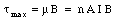## Solutions Homework Set # 7 - Physics 122

Problem 1

The total dipole moment of the coil is equal to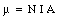where A is the cross sectional area of the coil. The torque associated with the magnetic forces, with respect to O, is equal to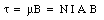Note: here we have used the fact that the dipole moment and the magnetic field are perpendicular to each other, and the parallel axis theorem. If the system is at rest with respect to O than the total torque must be equal to 0. The magnitude of the torque with respect to O due to the additional weight [Delta]M put on the scale is equal to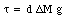where d is the distance between O and A. Since the total torque must be equal to zero we conclude that: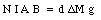or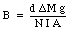Problem 2

The current through both resistors will not be equal if their resistance is different. The ratio of the current through resistor 1 and the current through resistor 2 is equal to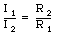or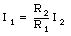Since the total current is given, we can calculate the current I1 and I2: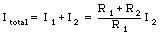and thus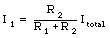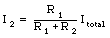The forces acting on the two current segments (see Figure) are equal to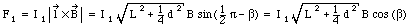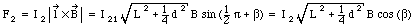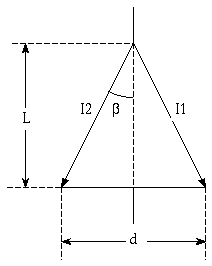Figure Problem 2

The torque with respect to the rotation axis due to these two forces is equal to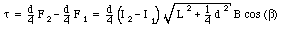Eliminating the angle [beta] from the last equation we obtain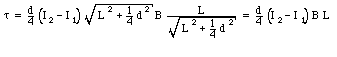The torque on the current loop is thus equal to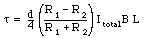Problem 3

Consider the situation shown in the Figure.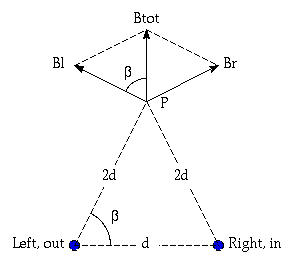Figure Problem 3.

The magnitude of the magnetic fields at P due to the left and right wires are equal in magnitude: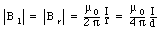The total magnetic field at P is the vector sum of these fields and is equal to: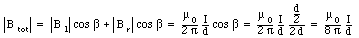Problem 4

When the cork ball moves in a magnetic field it experiences a force the produces circular motion. The period of this motion is equal to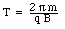During this time it changes its direction by 2[pi] radians. The problems provide you with information on the rate of change in direction. Based on the provided information, [Delta][theta] and [Delta]t, we can calculate the period T: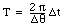Combing these two expressions for T we can conclude that: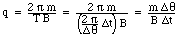Problem 5

Since the system is in equilibrium, the net torque must be equal to zero. The torque associated with the two masses is equal to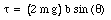The torque associated with the current loop is equal toSince the net torque must be equal to zero we conclude that: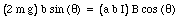This equation can be solved for [theta]: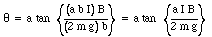Problem 6

The magnetic field at P can be calculated using the principle of superposition. The calculation of the magnetic field due to each side of the square is similar to the calculation of the magnetic field due to an infinitely long current wire, except that the integration limits of the angle are different (see Example 3 in Chapter 30, and the Figure below).Figure Problem 6

The contribution from the top and bottom segment are equal. Each contributed: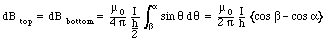The angles used in this equation are indicated in the Figure. The contributions from the left and the right segments are equal to: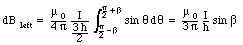and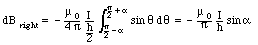The total magnetic field at P is thus equal to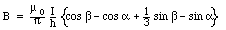The angles are equal to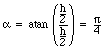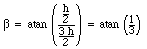Problem 7

The potential difference [Delta]V across the sample is equal to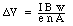where w is the width of the metal, B is the external magnetic field, I is the current though the metal, n is the density of charge carriers, and A is the cross sectional area of the metal. The cross sectional area A is equal to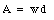where d is the thickness of the material. Thus: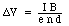The density of charge carriers can now be calculated on the basis of the measured quantities: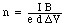Problem 8

The magnetic moment of the coil is equal to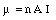where A is the area of the coil. The torque exerted on the coil by the magnetic field is equal to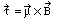The torque has a maximum value when the magnetic field is perpendicular to the magnetic moment. Thus: